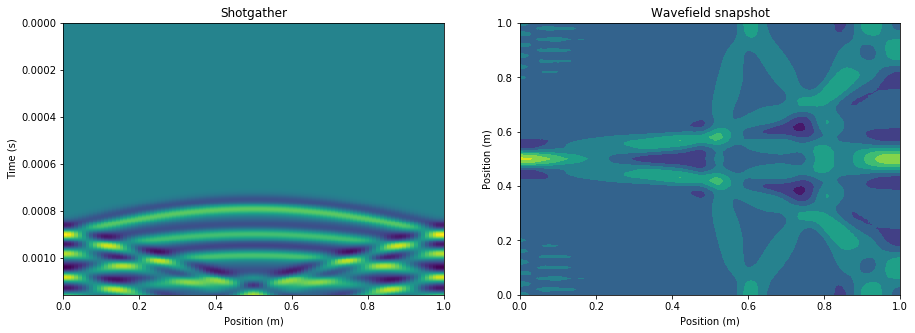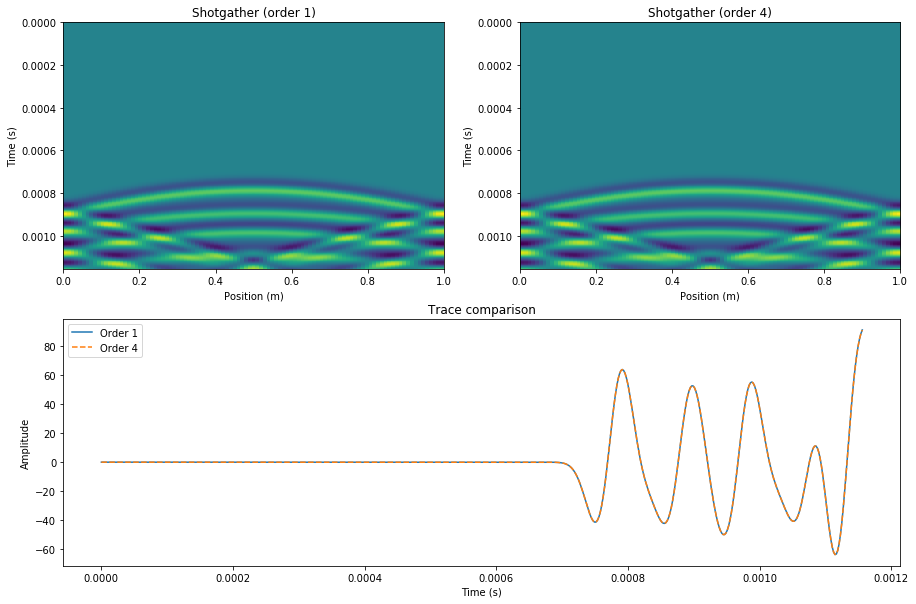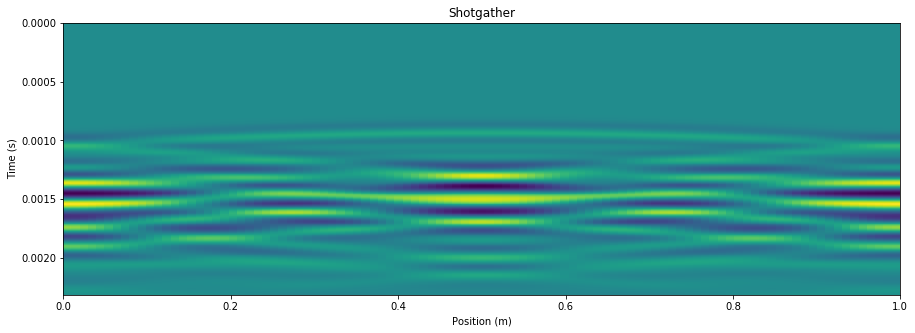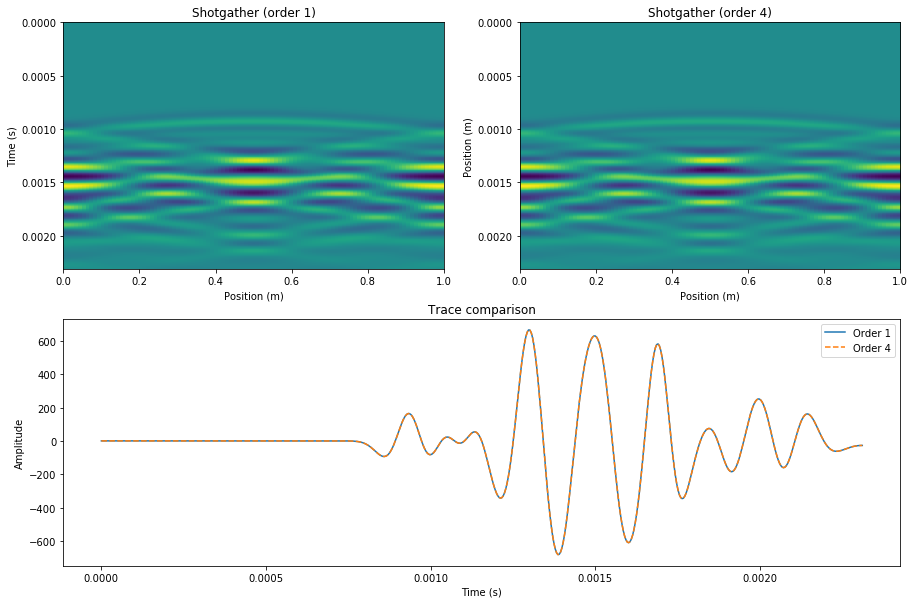# Model interpolation order

In this notebook we'll explore the concept of "model interpolation order". The spectral-element method works by approximating the dynamic field variables as Lagrange polynomials with time-varying coefficients. These polynomials are defined using the Gauss-Lobatto-Legendre (GLL) collocation points defined within each element. For a polynomial interpolation order of degree 4 (the default in Salvus), there will be 5 GLL points along each edge of a given element, which results in 25 and 125 points each for 2-D and 3-D elements, respectively. In general, a $d$-dimensional spectral element equipped with order $n$ interpolating polynomials will have $(n+1)^d$ GLL points in its interior.

While the GLL basis is the canonical method for representing the wavefield, we can also use it to represent the material parameters and spatial geometry. This tutorial shows how one can use different order (1, 2, and 4) interpolating polynomials to represent the model in Salvus. Specifically, we'll:

• Discretize a linearly-varying 2-D model onto 1st and 4th order polynomial bases
• Compare the wavefield solutions generated in each of these cases.
• Do the same in 3-D.

As always, the first step is to import our Python toolbox. Make sure you've gotten in from here.

Copy
%matplotlib inline
%config Completer.use_jedi = False

from functools import partial
from pathlib import Path

import h5py
import matplotlib.pyplot as plt
import numpy as np

import salvus_mesh.structured_grid_2D as sg2d
import salvus_mesh.structured_grid_3D as sg3d
import salvus_mesh.unstructured_mesh as um
import salvus_toolbox.toolbox as st
from salvus_flow import api
from salvus_flow import simple_config as sc

ranks = 2

## Setting up the models

First we set up a simple function to return basic meshes for testing in both 2 and 3 dimensions. The size of the mesh is small as these examples will only run for a trivial duration, and are just meant to be used to explore the possible parameterizations. We'll also set up a constant density throughout the domain.

n = 25
x = 1.0
rho = 1000.0
mesh = sg2d.StructuredGrid2D.rectangle(
nelem_x=n, nelem_y=n, max_x=x, max_y=x
).get_unstructured_mesh()
mesh.find_side_sets()

Now we copy the mesh skeleton we created above into two separate meshes, each of which we'll set to a different model interpolation order. For brevity we'll use here orders 1 and 4 (order 2 is also supported and the workflow is identical).

mesh_order_1 = mesh.copy()
mesh_order_1.change_tensor_order(1)

mesh_order_4 = mesh.copy()
mesh_order_4.change_tensor_order(4)

Now we'll copy the coordinate arrays for use with the interpolation function we will write below. Note that we randomly choose which axis to interpolate over.

axis = np.random.choice([0, 1])
coords_order_1 = mesh_order_1.get_element_nodes()[:, :, axis].flatten()
coords_order_4 = mesh_order_4.get_element_nodes()[:, :, axis].flatten()

The function below will linearly interpolate a range of model parameters over the coordinate range of a mesh.

def li(x: np.ndarray, min_val, max_val) -> np.ndarray:
"""Linearly interpolate a parameter over a coordinate array.

Parameters
----------
x : np.ndarray
Flattened coordinate array (either x or y).
min_val : float
Value of the parameter at the minimum coordinate.
max_val : float
Value of the parameter at the maximum coordinate.

Returns
-------
np.ndarray
An array of values linearly interpolated over the coordinate range.

"""
m = (max_val - min_val) / (np.max(x) - np.min(x))
return m * x + min_val

We'll use the linear $M0$ and $M1$ to parameterize our acoustic domain. For more information on different supported parameterizations in Salvus, please refer to the relevant tutorial.

vp0, vp1 = 1000, 2000
m10 = m11 = 1 / rho
m00 = m10 / vp0 ** 2
m01 = m11 / vp1 ** 2

Now we interpolate and attach the desired parameter to our 1st order mesh...

m0 = (np.apply_along_axis(li, 0, coords_order_1, m00, m01)).reshape(
mesh_order_1.nelem, mesh_order_1.nodes_per_element
) * 0.5

m1 = (np.apply_along_axis(li, 0, coords_order_1, m10, m11)).reshape(
mesh_order_1.nelem, mesh_order_1.nodes_per_element
) * 0.5

mesh_order_1.attach_field("M0", m0)
mesh_order_1.attach_field("M1", m1)
mesh_order_1.attach_field("fluid", np.ones(mesh.nelem))
mesh_order_1
When run interactively in a Jupyter Notebook, this output cell contains a widget. Click the button below to load a preview of it. Please note that most interactive functionality does not work without a running Python kernel.
<salvus_mesh.unstructured_mesh.UnstructuredMesh at 0x7ff5c304dba8>

...and our 4th order mesh.

m0 = (np.apply_along_axis(li, 0, coords_order_4, m00, m01)).reshape(
mesh_order_4.nelem, mesh_order_4.nodes_per_element
) * 0.5

m1 = (np.apply_along_axis(li, 0, coords_order_4, m10, m11)).reshape(
mesh_order_4.nelem, mesh_order_4.nodes_per_element
) * 0.5

mesh_order_4.attach_field("M0", m0)
mesh_order_4.attach_field("M1", m1)
mesh_order_4.attach_field("fluid", np.ones(mesh.nelem))

mesh_order_4
When run interactively in a Jupyter Notebook, this output cell contains a widget. Click the button below to load a preview of it. Please note that most interactive functionality does not work without a running Python kernel.
<salvus_mesh.unstructured_mesh.UnstructuredMesh at 0x7ff5c472b8d0>

Note here how both meshes end up do looking the same! This is because, no matter which interpolation order we choose, Salvus must regardless interpolate the material parameters from the grid defining the model interpolation to the grid defining the wavefield interpolation. This interpolation itself is a bilinear operation which, for the linear function we've chosen to interpolate, will return the same results (to numerical precision) as simply doing the interpolation oneself. Nevertheless, this is our point here as we want to show that the generated solutions are also the same. For an application where higher-order models make a significant different in the solution, check our the Marmousi tutorial in the "exploration" section.

## Setting up a simulation (order 1)

Now we'll set up some sources and receivers as is standard. Here we're mocking a "cross-hole" setup, where we have a source in one borehole and a line of receivers in another.

# Source in the left borehole.
src = sc.source.cartesian.ScalarPoint2D(
x=0.1, y=0.5, f=1, source_time_function=sc.stf.Ricker(center_frequency=1e4)
)

# String of receivers in the right borehole.
y=np.linspace(0, 1, 101),
side_set_name="x1",
station_code="xx",
fields=["phi"],
offset=-0.1,
)

w1 = sc.simulation.Waveform(mesh=mesh_order_1, sources=src, receivers=recs)
/miniconda/envs/py37/lib/python3.7/site-packages/salvus_flow/simple_config/simulation/__init__.py:218: UserWarning: If you install the joblib module, the src/rec location process would run in parallel.
cpu_count=cpu_count,


Now we just need to set a few more parameters and we're good to go.

# Output receivers in simplified HDF5 format.
w1.output.point_data.format = "hdf5"

# Run the simulation long enough that wave hit the receivers.
w1.physics.wave_equation.end_time_in_seconds = 1e-3

# Output the state variable at all time steps (for comparison).
w1.output.volume_data.format = "hdf5"
w1.output.volume_data.fields = ["phi"]
w1.output.volume_data.filename = "output.h5"
w1.output.volume_data.sampling_interval_in_time_steps = 1

# Validate and plot.
w1.validate()
w1
When run interactively in a Jupyter Notebook, this output cell contains a widget. Click the button below to load a preview of it. Please note that most interactive functionality does not work without a running Python kernel.
<salvus_flow.simple_config.simulation.Waveform object at 0x7ff5be7345f8>

Everything looks good, so let's now go ahead and run the simulation on our order 1 model.

# Output folder 1.
of1 = Path("output_order_1")

# Run.
api.run(
ranks=ranks,
get_all=True,
input_file=w1,
overwrite=False,
site_name="local",
output_folder=of1,
)
Job job_1910230021486435_77dede027c running on local with 2 rank(s).
Site information:
* Salvus version: 0.10.6
* Floating point size: 32
-> Current Task: Time loop complete* Downloaded 43.8 MB of results to output_order_1.
* Total run time: 0.98 seconds.
* Pure simulation time: 0.63 seconds.

<salvus_flow.sites.salvus_job.SalvusJob at 0x7ff5be4ce9e8>

Now let's see some quick plots to see how everything looks.

# Get output data into notebook.
f, ax = plt.subplots(1, 2, figsize=(15, 5))
tri, d1_vol = st.visualize_wavefield_2d(of1 / "output.h5", field="phi")
d1, t, extent = st.get_shotgather(of1 / "receivers.h5", field="phi", axis=1)

# Plot shotgather.
ax.imshow(d1, extent=extent, aspect="auto")
ax.set_xlabel("Position (m)")
ax.set_ylabel("Time (s)")
ax.set_title("Shotgather")

# Plot last time step of wavefield.
ax.tricontourf(tri, d1_vol[-1, :])
ax.set_xlabel("Position (m)")
ax.set_ylabel("Position (m)")
ax.set_title("Wavefield snapshot")
Text(0.5, 1.0, 'Wavefield snapshot')Looks as we might expect. Now, moving onto order 4.

## Setting up a simulation (order 4)

All we need to do here is initialize a new simulation object for our order 4 mesh. We can re-use the sources and receivers which we've created above.

w4 = sc.simulation.Waveform(mesh=mesh_order_4, sources=src, receivers=recs)
/miniconda/envs/py37/lib/python3.7/site-packages/salvus_flow/simple_config/simulation/__init__.py:218: UserWarning: If you install the joblib module, the src/rec location process would run in parallel.
cpu_count=cpu_count,


Again, setting some output parameters so we can analyze the solution...

w4.output.point_data.format = "hdf5"
w4.physics.wave_equation.end_time_in_seconds = 1e-3

w4.output.volume_data.format = "hdf5"
w4.output.volume_data.fields = ["phi"]
w4.output.volume_data.filename = "output.h5"
w4.output.volume_data.sampling_interval_in_time_steps = 1

w4.validate()

...and running the simulation.

# Output folder 4.
of4 = Path("output_order_4")

# Run.
api.run(
input_file=w4,
site_name="local",
ranks=ranks,
output_folder=of4,
overwrite=False,
get_all=True,
)
Job job_1910230021788246_5b18887d7c running on local with 2 rank(s).
Site information:
* Salvus version: 0.10.6
* Floating point size: 32


* Downloaded 43.8 MB of results to output_order_4.
* Total run time: 2.95 seconds.
* Pure simulation time: 1.61 seconds.

<salvus_flow.sites.salvus_job.SalvusJob at 0x7ff5be1f6390>

Now we can look at the solution and see that indeed the same solution has been generated! Keep in mind though that there are some theoretical caveats to using model parameterizations which vary quickly within each element. For more information on these caveats please see the reference manual and the associated scientific papers.

f = plt.figure(figsize=(15, 10))

d1, dt, extent = st.get_shotgather(of1 / "receivers.h5", field="phi", axis=1)
d4, dt, extent = st.get_shotgather(of4 / "receivers.h5", field="phi", axis=1)

ax0.imshow(d1, extent=extent, aspect="auto")
ax0.set_xlabel("Position (m)")
ax0.set_ylabel("Time (s)")
ax0.set_title("Shotgather (order 1)")

ax1.imshow(d4, extent=extent, aspect="auto")
ax1.set_xlabel("Position (m)")
ax1.set_ylabel("Time (s)")
ax1.set_title("Shotgather (order 4)")

ax2.set_title("Trace comparison")
ax2.plot(np.arange(d4.shape) * dt, d1[:, 50], label="Order 1")
ax2.plot(np.arange(d4.shape) * dt, d4[:, 50], label="Order 4", ls="dashed")
ax2.set_xlabel("Time (s)")
ax2.set_ylabel("Amplitude")
ax2.legend()
<matplotlib.legend.Legend at 0x7ff5be5f7a58># Testing, removed for tutorial.
np.testing.assert_allclose(d1, d4, atol=1e-5 * np.max(np.abs(d1)))

## 3D

For completeness we also show how different model orders can be used to parameterize 3D models. To ensure a quick run time, we'll use a smaller number of elements in each dimension.

# Generate mesh w/ 10 elements per dim.
n = 10
mesh = sg3d.StructuredGrid3D.cube(
nelem_x=n, nelem_y=n, nelem_z=n, max_x=x, max_y=x, max_z=x
).get_unstructured_mesh()
mesh.find_side_sets()
# Copy into two different meshes and tensorize.
mesh_order_1 = mesh.copy()
mesh_order_1.change_tensor_order(1)

mesh_order_4 = mesh.copy()
mesh_order_4.change_tensor_order(4)
# Choose an axis to interpolate over.
axis = np.random.choice([0, 1, 2])
coords_order_1 = mesh_order_1.get_element_nodes()[:, :, axis].flatten()
coords_order_4 = mesh_order_4.get_element_nodes()[:, :, axis].flatten()
# Interpolate the models (order 1).
m0 = (np.apply_along_axis(li, 0, coords_order_1, m00, m01)).reshape(
mesh_order_1.nelem, mesh_order_1.nodes_per_element
) * 0.5

m1 = (np.apply_along_axis(li, 0, coords_order_1, m10, m11)).reshape(
mesh_order_1.nelem, mesh_order_1.nodes_per_element
) * 0.5

mesh_order_1.attach_field("M0", m0)
mesh_order_1.attach_field("M1", m1)
mesh_order_1.attach_field("fluid", np.ones(mesh.nelem))
mesh_order_1
When run interactively in a Jupyter Notebook, this output cell contains a widget. Click the button below to load a preview of it. Please note that most interactive functionality does not work without a running Python kernel.
<salvus_mesh.unstructured_mesh.UnstructuredMesh at 0x7ff5be6fabe0>
# Interpolate the models (order 4).
m0 = (np.apply_along_axis(li, 0, coords_order_4, m00, m01)).reshape(
mesh_order_4.nelem, mesh_order_4.nodes_per_element
) * 0.5

m1 = (np.apply_along_axis(li, 0, coords_order_4, m10, m11)).reshape(
mesh_order_4.nelem, mesh_order_4.nodes_per_element
) * 0.5

mesh_order_4.attach_field("M0", m0)
mesh_order_4.attach_field("M1", m1)
mesh_order_4.attach_field("fluid", np.ones(mesh.nelem))

mesh_order_4
When run interactively in a Jupyter Notebook, this output cell contains a widget. Click the button below to load a preview of it. Please note that most interactive functionality does not work without a running Python kernel.
<salvus_mesh.unstructured_mesh.UnstructuredMesh at 0x7ff5be6fac88>
# Set up a simulation.

# Source in the left borehole.
src = sc.source.cartesian.ScalarPoint3D(
x=0.1,
y=0.5,
z=0.5,
f=1,
source_time_function=sc.stf.Ricker(center_frequency=5e3),
)

# String of receivers in the right borehole.
recs = [
x=0.9, y=0.5, z=z, station_code=f"{i:03d}", fields=["phi"]
)
for i, z in enumerate(np.linspace(0, 1, 101))
]

w1 = sc.simulation.Waveform(mesh=mesh_order_1, sources=src, receivers=recs)
# Finalize simulation setup.
# Output receivers in simplified HDF5 format.
w1.output.point_data.format = "hdf5"

# Run the simulation long enough that wave hit the receivers.
w1.physics.wave_equation.end_time_in_seconds = 2e-3

# Output the state variable at all time steps (for comparison).
w1.output.volume_data.format = "hdf5"
w1.output.volume_data.fields = ["phi"]
w1.output.volume_data.filename = "output.h5"
w1.output.volume_data.sampling_interval_in_time_steps = 1

# Validate and plot.
w1.validate()
w1
When run interactively in a Jupyter Notebook, this output cell contains a widget. Click the button below to load a preview of it. Please note that most interactive functionality does not work without a running Python kernel.
<salvus_flow.simple_config.simulation.Waveform object at 0x7ff5be66ccc0>
# Run simulation.
of1 = Path("output_order_1")

# Run.
api.run(
ranks=ranks,
get_all=True,
input_file=w1,
overwrite=True,
site_name="local",
output_folder=of1,
)
Job job_1910230021779959_42831ef297 running on local with 2 rank(s).
Site information:
* Salvus version: 0.10.6
* Floating point size: 32


* Downloaded 286.2 MB of results to output_order_1.
* Total run time: 3.34 seconds.
* Pure simulation time: 1.76 seconds.

<salvus_flow.sites.salvus_job.SalvusJob at 0x7ff5be6fa6a0>
# Visualize shotgather.
f, ax = plt.subplots(1, 1, figsize=(15, 5))
d1, t, extent = st.get_shotgather(of1 / "receivers.h5", field="phi", axis=2)

# Plot shotgather.
ax.imshow(d1, extent=extent, aspect="auto")
ax.set_xlabel("Position (m)")
ax.set_ylabel("Time (s)")
ax.set_title("Shotgather")
Text(0.5, 1.0, 'Shotgather')# Do the same for order 4.

w4.output.point_data.format = "hdf5"
w4.physics.wave_equation.end_time_in_seconds = 2e-3

w4.output.volume_data.format = "hdf5"
w4.output.volume_data.fields = ["phi"]
w4.output.volume_data.filename = "output.h5"
w4.output.volume_data.sampling_interval_in_time_steps = 1

w4.validate()
# Output folder 4.
of4 = Path("output_order_4")

# Run.
api.run(
input_file=w4,
site_name="local",
ranks=ranks,
output_folder=of4,
overwrite=True,
get_all=True,
)
Job job_1910230021401940_5a9314a1d2 running on local with 2 rank(s).
Site information:
* Salvus version: 0.10.6
* Floating point size: 32


* Downloaded 286.2 MB of results to output_order_4.
* Total run time: 4.14 seconds.
* Pure simulation time: 2.60 seconds.

<salvus_flow.sites.salvus_job.SalvusJob at 0x7ff5bba6b0b8>
# Plot and compare results.
f = plt.figure(figsize=(15, 10))

d1, dt, extent = st.get_shotgather(of1 / "receivers.h5", field="phi", axis=2)
d4, dt, extent = st.get_shotgather(of4 / "receivers.h5", field="phi", axis=2)

ax0.imshow(d1, extent=extent, aspect="auto")
ax0.set_xlabel("Position (m)")
ax0.set_ylabel("Time (s)")
ax0.set_title("Shotgather (order 1)")

ax1.imshow(d4, extent=extent, aspect="auto")
ax1.set_xlabel("Position (m)")
ax1.set_ylabel("Position (m)")
ax1.set_title("Shotgather (order 4)")

ax2.set_title("Trace comparison")
ax2.plot(np.arange(d1.shape) * dt, d1[:, 50], label="Order 1")
ax2.plot(np.arange(d4.shape) * dt, d4[:, 50], label="Order 4", ls="dashed")
ax2.set_xlabel("Time (s)")
ax2.set_ylabel("Amplitude")
ax2.legend()
<matplotlib.legend.Legend at 0x7ff5bba046a0>PAGE CONTENTS